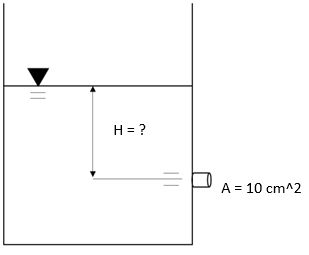## Discharging Orifice

Consider the shown water tank with a nozzle cross-sectional area of 10 cm^2 and a discharge velocity of 5 m/s. Assuming there are no minor losses in the discharge and a common coefficient of discharge of 0.2, what is the water line’s height from the nozzle?Hint
$$Q=CA(2gh)^{1/2}$$$where $$Q$$ is the volumetric flow rate, $$C$$ is the coefficient of discharge, $$A$$ is the cross sectional area of flow, $$g$$ is the acceleration due to gravity, and $$h$$ is the height of the fluid above the orifice. Hint 2 $$Q=vA$$$
where $$Q$$ is the volumetric flow rate, $$v$$ is the fluid’s velocity, and $$A$$ is the cross sectional area of flow.
For an orifice discharging fluid freely into the atmosphere:
$$Q=CA(2gh)^{1/2}$$$where $$Q$$ is the volumetric flow rate, $$C$$ is the coefficient of discharge, $$A$$ is the cross sectional area of flow, $$g$$ is the acceleration due to gravity, and $$h$$ is the height of the fluid above the orifice. Since $$Q=vA$$ where $$v$$ is the fluid’s velocity and $$A$$ is the cross sectional area of flow, it’s possible to combine the two equations into one and rearrange to solve for height: $$vA=CA(2gh)^{1/2}$$$
$$v=C(2gh)^{1/2}=(0.2)\sqrt{2(9.8m/s^2)}(h)^{0.5}=5m/s$$$$$\sqrt{2(9.8m/s^2)h}=\frac{5m/s}{0.2}=25m/s$$$
$$2(9.8m/s^2)h=(25m/s)^2=625m^2/s^2$$$$$h=\frac{625m}{2(9.8)}=31.89m=32\:m$$$
32 m